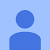# Simple Matrix Factorization example on the Movielens dataset using Pyspark

Matrix factorization works great for building recommender systems. I think it got pretty popular after the Netflix prize competition. All you need to build one is information about which user bought or rated which items and you’re good to go. And I was surprised how amazingly simple to build one with Pyspark ML libraries. So I’ll demonstrate how to code one up quickly using RDDs and DataFrames separately.

I’ll mostly focus on building the model in this tutorial. If you’re interested in learning more about Matrix factorization or Singular Value Decomposition, there are some amazing resources out there. And other than recommender systems, it also has applications in dimensionality reduction.

I’ll use the famous Movielens 1 million dataset. You can get it from here. Lets get started.

`# importing some librariesimport numpy as npimport pandas as pdimport pysparkfrom pyspark.sql import SQLContextsqlContext = SQLContext(sc)# check if spark context is definedprint(sc.version)`

First I’ll build the model using RDDs. I’d suggest you go with the DataFrame API and I’ll cover that later.

`# importing the MF librariesfrom pyspark.mllib.recommendation import ALS, \                            MatrixFactorizationModel, Rating# reading the movielens datadf_rdd = sc.textFile('ml-1m/ratings.dat')\            .map(lambda x: x.split("::"))`

Im reading the data as an rdd. The ratings has four columns — user, item, rating, timestamp. We dont need the timestamp. Let me get rid of it and get our data ready to be fed into the model.

`ratings= df_rdd.map(lambda l:\            Rating(int(l),int(l),float(l)))`

I’ll split the data into train and test sets. And train the model.

`X_train, X_test= ratings.randomSplit([0.8, 0.2])# Training the modelrank = 10numIterations = 10model = ALS.train(X_train, rank, numIterations)`

Here, ALS stands for Alternate Least Squares, which is the name of the optimization algorithm used for Matrix Factorization. You can add more parameters before you train the model — like if you’d like some regularization or youd want the final two matrix to have positive values. Finally lets see how good our mode is on the test data.

`# Evaluate the model on testdata# dropping the ratings on the tests datatestdata = X_test.map(lambda p: (p, p))predictions = model.predictAll(testdata).map(lambda r: ((r, r), r))# joining the prediction with the original test datasetratesAndPreds = X_test.map(lambda r: ((r, r), r)).join(predictions)# calculating errorMSE = ratesAndPreds.map(lambda r: (r - r)**2).mean()print("Mean Squared Error = " + str(MSE))`

Done. Now lets do the same thing with DataFrames. I’ll need some more libraries for this.

`# importing ALS from pyspark.ml which works on Dataframesfrom pyspark.ml.recommendation import ALS # Things we'll use for evaluationfrom pyspark.sql.types import FloatTypefrom pyspark.ml.evaluation import RegressionEvaluatorfrom pyspark.sql.functions import col# reading data as rdd and converting into a dataframedf = sc.textFile('deep-learning/ml-1m/ratings.dat.filepart')\      .map(lambda x: x.split("::")).toDF(["user", "item",\                                        "rating","timestamp"])# splitting into train and test setsX_train, X_test = df.randomSplit([0.6, 0.4])`

The syntax is a bit different when youre dealing with dataframes. But it acts the same way. I think you have to name the columns exactly as “user”, “item” and “rating” else it throws an error.

`# training the modelals = mlALS(rank=5, maxIter=10, seed=0)model = als.fit(X_train.select(["user", "item", "rating"]))`

Now, to get the predictions you can feed just the user and item columns from the test dataset and youll get back the predicted ratings joined with the original data.

`predictions =model.transform(X_test.select(["user", "item"]))`

Now Ill calculate the error. Before they’re passed onto the RegressionEvaluator(), the y_true and y_pred needs to be lab label and raw.

`# joining the predictions with the original tableratesAndPreds = X_test.join(predictions,(X_test.user == \    predictions.user) & (X_test.item == predictions.item) ,\           how='inner').select(X_test.user,X_test.item, \                 X_test.rating, predictions.prediction)# renaming the columns as raw and labelratesAndPreds = ratesAndPreds.select([col("rating").alias("label"),\                 col('prediction').alias("raw")])\                 ratesAndPreds = ratesAndPreds.withColumn("label", \                 ratesAndPreds["label"].cast(FloatType()))# calculate the errorevaluator = RegressionEvaluator(predictionCol="raw")evaluator.evaluate(ratesAndPreds2, {evaluator.metricName: "mae"})`

There are other handy methods like recommendForAllUsers() and recommendForAllItems() which gives top items and user per user or item which you can try out.

Thats it. Thanks for making it to the end. If this was helpful leave a like and a comment.

--

--

--

## More from Soumya Ghosh

Love podcasts or audiobooks? Learn on the go with our new app.## Did Stacking Improve My PySpark Churn Prediction Model?## Churn Prediction with PySpark## How to use PySpark Streaming with Google Colaboratory## Recommendation System with Content-based Filtering